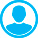SOME LINEAR AND NONLINEAR EQUATIONS OF HIGHER ORDERAbstract (English):
In this article we consider boundary value problems for some linear and nonlinear differential equations with partial derivatives of the sixth, fifth, fourth and third orders. We write out conditions on equation coefficients for which existence and uniqueness of solutions from Sobolev's space occur. If these conditions on equation coefficients are not valid, then there are given examples when solution is not unique, or is not unstable, or does not belong to Sobolev's space from existence and uniqueness theorem even for analytical coefficients and analytical right side of differential equation. After S.P. Novikov’s fundamental study in 1974 the interest to the nonlinear Korteweg-de Vries equation, Kadomtsev-Petviashvili equation and other nonlinear equations significantly grew. In this study of such equations we used methods of algebraic geometry integration and expansion method. In these studies exact solutions of special nonlinear equations series in partial derivatives play a big role. Solvability of similar equations was also studied in articles of A.I. Kozhanov, N.A. Larkin and other authors. The aim of this article is to find some exact solutions for special series partial differential equations. Solution graphs of such problems for linear equations and for the Korteweg-de Vries, Burgers-Korteweg-deVries, and Kadomtsev-Petviashvili equations are constructed.

Keywords:
linear and nonlinear equations, Korteweg-de Vries equation, boundary conditions, non-uniqueness of solution, instability of solution, graphs of solutions

1. Vragov V.N. Kraevye zadachi dlya neklassicheskikh uravneniy matematicheskoy fiziki [Boundary value problems for nonclassical equations of mathematical physics]. Novosibirsk: Novosibirsk University, 1983. 90 p.

2. Demidenko G.V., Uspenskii S.V. Uravneniya i sistemy, ne razreshennye otnositel'no starshey proizvodnoy [Equations and systems are not permitted with respect to the highest derivative]. Novosibirsk Scientific Book, 1998. 438 p.

3. Egorov I. E., Pyatkov S. G., Popov S.V. Neklassicheskie differentsial'no-operatornye uravneniya [Nonclassical differential-operator equations]. Novosibirsk: Publishing House of Science, 2000. 336 p.

4. Kozhanov A.I. Nachal'no-kraevaya zadacha dlya uravneniya tipa obobshchennogo uravneniya Busineska s nelineynym istochnikom [Initial boundary value problem for the generalized Boussinesq equation type with a nonlinear source]. Mat. Deputy, 1999, vol. 65, no. 1, pp. 70-75.

5. Chueshev A.V. Ob odnom nelineynom uravnenii smeshannogo tipa nechetnogo poryadka [On a nonlinear equation of mixed type of odd order]. Vestnik Novosib. un-ta, seriya "matematika, mekhanika, informatika" [Bulletin Novosib. University, series "Mathematics, Mechanics and Computer Science"]. Novosibirsk, 2001, vol. 1, no. 1, pp. 107-123.

6. Chuesheva N. A. Ob odnoy kraevoy zadache dlya nelineynogo uravneniya tret'ego poryadka [On a boundary value problem for nonlinear third-order equations]. Differentsial'nye uravneniya [Differential Equations]. Minsk, 1980, vol. 16, no. 1, pp. 183-185.

7. Chuesheva N. A., Maksimova E.V. Kraevaya zadacha dlya differentsial'nogo uravneniya chetvertogo poryadka [Boundary value problem for the fourth-order differential equation]. Vestnik KemGU [Bulletin of Kemerovo State University]. Kemerovo, 2011, no. 3/1, pp. 266-268.

8. Chuesheva N. A. Neskol'ko lineynykh i nelineynykh differentsial'nykh uravneniy vysokogo poryadka [Some linear and nonlinear differential equations of higher order]. Dni geometrii v Novosibirske tez. mezhdunar. konf. Novosibirsk, 26-29 avgusta [Days of geometry in Novosibirsk: mes. Intern. Conf.], Novosibirsk, 26-29 of August. Novosibirsk Institute of Mathematics named after S.B. Sobolev. Novosibirsk, 2015, pp. 67-68.

9. Chuesheva N. A. Kraevye zadachi dlya nekotorykh uravneniy tret'ego poryadka [Boundary problems for some of the third-order differential equations]. Dni geometrii v Novosibirske: tez. mezhdunar. konf., Novosibirsk, 24-27 sentyabrya [Days of geometry in Novosibirsk: mes. Intern. Conf., Novosibirsk, 24-27 of September.] Novosibirsk Institute of Mathematics named after S.B. Sobolev. Novosibirsk, 2014, pp. 80-81.

10. Takamori Kato. Well-posedness for the fifth order KdV equation. Funkc. Ekvacioj=Funct. Equat., 2012, vol. 55, no. 1, pp. 17-53.

11. Shu Wen. The Cauchy equation of the Degaspersive - Procesi equation with the dispersive term. Nonlinear Sci, 2008, vol. 5, no. 3, pp. 210-216.

12. Inan I. E. -Expansion Method for Traveling Wave Solutions of the Sixth-Order Ramani Equation. Cankaya University Journal of Science and Engineering, 2010, vol. 7, no. 1, pp. 51-57.

13. Chuesheva N.A. Neskol'ko lineynykh i nelineynykh differentsial'nykh uravneniy [Some linear and nonlinear differential equations]. Dni geometrii v Novosibirske: tez. mezhdunar. konf., Novosibirsk, 28-31 avgusta [Days of geometry in Novosibirsk: mes. Intern. Conf., Novosibirsk, 28-31 of August]. Novosibirsk, Novosibirsk Institute of Mathematics named after S.B. Sobolev, 2013, pp. 93-94.